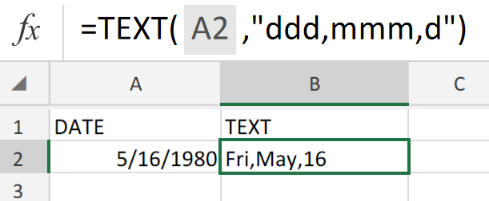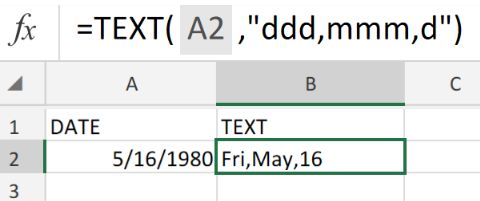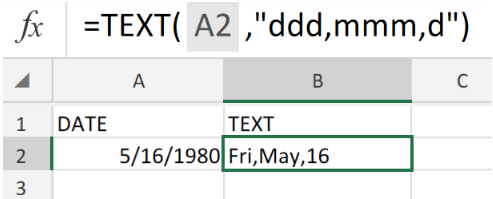Get instant live expert help with Excel or Google Sheets“My Excelchat expert helped me in less than 20 minutes, saving me what would have been 5 hours of work!”

#### Post your problem and you’ll get expert help in seconds.

Your message must be at least 40 characters
Our professional experts are available now. Your privacy is guaranteed.

# Convert date to textFigure 1. Convert Date to Text.

If we want to convert a calendar date in its number format, to text format, we are going to utilize the Excel TEXT Function.

## Generic Formula

`=TEXT(date,format)`

• date is the number value we want to convert.
• format is the pattern in which we want the resulting text string to appear.

Time and dates are originally stored as serial numbers in Excel before they are transformed into values that can be read by us.

## How to use the Excel TEXT Function.

We will now demonstrate how we can use the Excel TEXT Function to convert a date in number format into text. .Figure 2. Convert Date to Text in Excel.

In the example illustrated above, our purpose is to convert the date in cell A2 to a text string format.

We entered the formula for the TEXT Function into cell B2.

The Excel TEXT Function returned the text string date format in cell B2.

The TEXT Function can be used for converting numeric values or dates in a fixed format.Figure 3. Final Result.

## Instant Connection to an Expert through our Excelchat Service:

Our live Excelchat Service is here for you. We have Excel Experts available 24/7 to answer any Excel questions you may have. Guaranteed connection within 30 seconds and a customized solution for you within 20 minutes

### Did this post not answer your question? Get a solution from connecting with the expert.Another blog reader asked this question today on Excelchat:
Solution examplesGood morning, I need a cell formatting solution to display leading zero in my excel table with the following conditions. 1. Cannot format as text 2. cell size is not fixed. Please let me know if there is a solution.Thanks in advance
Solved by V. C. in 11 minslooking for an Iferror formula that will bring back a blank value if a date is not listed. Currently using the following but want to add an Iferror statement: =TEXT(H2,"DDDD"). H2 is the raw date ex. 2/8/18. I tried =IFERROR(TEXT(H2,"DDDD"),"") but it keeps returning "saturday" instead of a blank cell. Any help is appreciated.
Solved by F. Q. in 20 minsHow do I make this formula =Value(IFerror(TEXT(AM3,"HMM"),"0")) Return a value of 2400 if the value is not an error?
Solved by F. J. in 21 minsI have an excel spreadsheet with two worksheets. And the following formula is not pulling the data. =VLOOKUP(\$A\$2:\$A\$566,'Module Type Info'!\$A\$2:\$D\$97,4,FALSE) In sheet 1, I am using all data in column 1 for the lookup In sheet 2, I have selected the first 4 columns of data for array I want to pull data from sheet 2, column 4 into sheet 1 Both tabs are sorted alphabetically. Confirmed that the value in column 4 of sheet 2 is a TEXT field. Why is this not pulling the info from sheet 2 into sheet 1
Solved by F. L. in 39 minsI need to add an formula into this one: =IF(\$L\$1="","",VLOOKUP(TEXT(\$L\$1,"000000"),'FRS102 BASE & GBP TB MAY 2018'!\$E:\$X,MATCH(A25,'FRS102 BASE & GBP TB MAY 2018'!\$Q\$3:\$X\$3,0)+3,0))
Solved by K. D. in 11 mins## Subscribe to Excelchat.coAnother blog reader asked this question today on Excelchat: